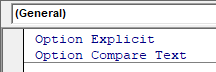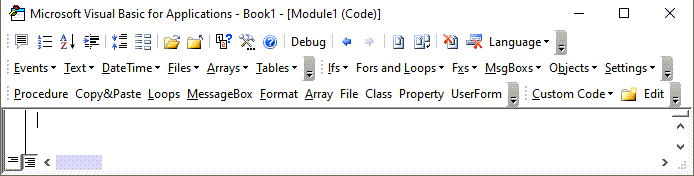# VBA Search for (Find) Value in Array

This tutorial will demonstrate how to Search for (Find) a Value in an Array in VBA

There are a number of ways you can search for a string in an array – depending on whether the array is a one dimensional or multi-dimensional.

## Searching in a One-Dimensional Array

To search for a value in a one-dimensional array, you can use the Filter Function.

``````Dim z As Variant
'filter the original array
z = Filter(Array, String, True, vbCompareBinary)``````

The Syntax of the Filter option is a follows

Filter(Source Array, Match as String, [Include as Boolean], [Compare as vbCompareMethod])

The Source Array and the Match as String are required while the Include as Boolean and the Compare as vbCompareMethod are optional.  If these are not included they are set to True and vbCompareBinary respectively.

### Find values that match the Filter

``````Sub FindBob()
'Create Array
Dim strName() As Variant
strName() = Array("Bob Smith", "John Davies", "Fred Jones", "Steve Jenkins", "Bob Williams")

'declare a variant to store the filter data in
Dim strSubNames  As Variant

'filter the original array
strSubNames = Filter(strName, "Bob")

'if you UBound value is greater than -1, then the value has been found
If UBound(strSubNames ) > -1 Then MsgBox ("I found Bob")
End Sub``````

The second array will hold the values found by the filter.  If your UBound values are not -1, then the array has managed to find the  value that you were searching for.

You can also see how many times the text appears in the original array.

``````Sub CountNames()
'Create array
Dim strName() As Variant
strName() = Array("Bob Smith", "John Davies", "Fred Jones", "Steve Jenkins", "Bob Williams")

'declare an array to store the filter data in
Dim strSubNames As Variant

'filter the original array
strSubNames = Filter(strName, "Bob")

'if you add 1 to the UBound value, we will get the number of times the text appears
Msgbox  UBound(strSubNames) + 1 & " names found."
End Sub``````

### Find values that DO NOT match the Filter

The [Include as Boolean] option allows you to find how many values in your array which DO NOT match your filter

``````Sub CountExtraNames()
'create array
Dim strName() As Variant
strName() = Array("Bob Smith", "John Davies", "Fred Jones", "Steve Jenkins", "Bob Williams")

'declare an array to store the filter data in
Dim strSubNames As Variant

'filter the original array
strSubNames = Filter(strName, "Bob", False)

'if you add 1 to the UBound value, we will get the number of times the text appears
Msgbox  UBound(strSubNames) + 1 & " names found."
End Sub``````

we have therefore amended this line:

``strSubNames = Filter(strName, "Bob")``

with this line:

``strSubNames = Filter(strName, "Bob", False)``

Using this line in the code, would return all the names that do NOT match “Bob”.

### Case Sensitive Filters

You will find that the filter is case sensitive by default.  This is true for all VBA functions.  If you want to search for text that is not case sensitive, you need to amend your code slightly.

``z = Filter(strName, "bob",, vbTextCompare)``

Adding vbTextCompare to your filter line will enable your code to find “bob” or “Bob”.  If this is omitted, VBA by default uses vbBinaryCompare which will only look for data that is an EXACT match.  Notice in the example above, we have left out the [Include as Boolean] argument so True is assumed.

### Option Compare Text

Alternatively, you can add the text Option Compare Text to the top of your module – this will make all the functions that you write in that particular module case insensitive.Stop searching for VBA code online. Learn more about AutoMacro - A VBA Code Builder that allows beginners to code procedures from scratch with minimal coding knowledge and with many time-saving features for all users!## Using a Loop to Search through an array

Using a loop is a little bit more complicated than using the Filter function.  We can create a function that will loop through all the values in the array.

``````Sub LoopThroughArray()
'create array
Dim strName() As Variant
strName() = Array("Bob Smith", "John Davies", "Fred Jones", "Steve Jenkins", "Bob Williams")

Dim strFind as string
strFind = "Bob"

Dim i As Long
'loop through the array
For i = LBound(strName, 1) To UBound(strName, 1)
If InStr(strName(i), strFind) > 0 Then
MsgBox "Bob has been found!"
Exit For
End If
Next i
End Sub``````

In order to find a part of the text string ie “Bob” instead of “Bob Smith” or “Bob Williams”, we needed to use the Instr Function in the If Statement.  This looked in the string returned by the loop from the Array to see if “Bob” was in the string, and as it was in the string, it would return a message box and then Exit the Loop.

## Searching in a Multi-Dimensional Array

We also use the loop to search through a multi-dimensional array.  Once again, we need to create a function than enables us to loop through all the values in the array, but this time, we also need to loop through each dimension of the array.

``````Function LoopThroughArray()
Dim varArray() As Variant
Dim strFind As String
strFind = "Doctor"
'declare the size of the array
ReDim varArray(1, 2)
'initialise the array
varArray(0, 0) = "Mel Smith"
varArray(0, 1) = "Fred Buckle"
varArray(0, 2) = "Jane Eyre"
varArray(1, 0) = "Accountant"
varArray(1, 1) = "Secretary"
varArray(1, 2) = "Doctor"
'declare variables for the loop
Dim i As Long, j As Long
'loop for the first dimension
For i = LBound(varArray, 1) To UBound(varArray, 1)
'loop for the second dimension
For j = LBound(varArray, 2) To UBound(varArray, 2)
'if we find the value, then msgbox to say that we have the value and exit the function
If varArray(i, j) = strFind Then
MsgBox "Doctor has been found!"
Exit Function
End If
Next j
Next i
End Function
``````